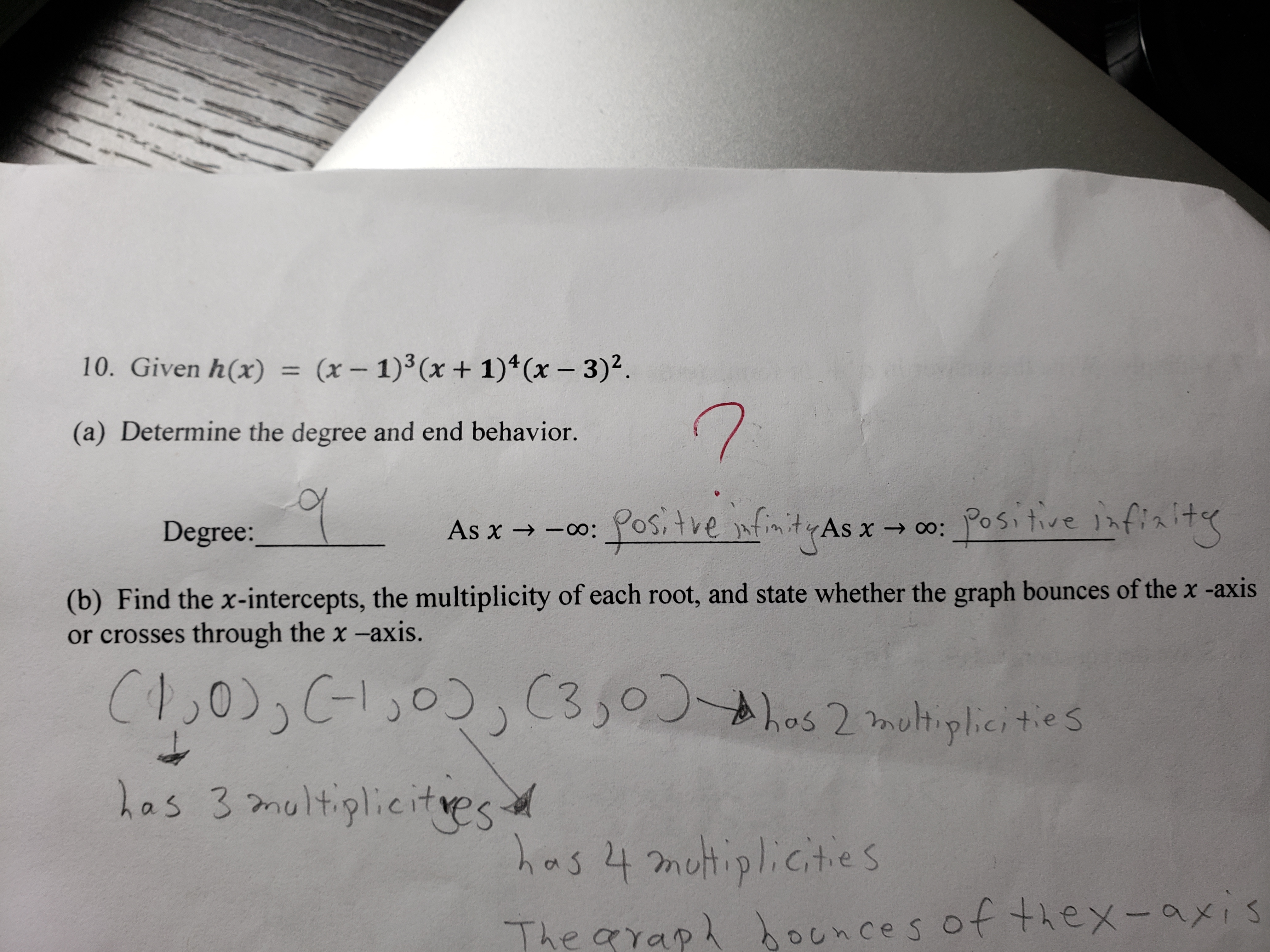10. Given h(x)(x 1)3 (x1)4 (x 3)2(a) Determine the degree and end behavior.As χ →_oo : yos, t ve ).f.m.t (As χ→ oo :posit-ve) hf7xitDegree:(b) Find the x-intercepts, the multiplicity of each root, and state whether the graph bounces of the x -axisor crosses through the x -axis.Los 3 molthiylietesThe grap ounces of thex-axis

Questionhelp_outlineImage Transcriptionclose10. Given h(x)(x 1)3 (x1)4 (x 3)2 (a) Determine the degree and end behavior. As χ →_oo : yos, t ve ).f.m.t (As χ→ oo : posit-ve ) hf7xit Degree: (b) Find the x-intercepts, the multiplicity of each root, and state whether the graph bounces of the x -axis or crosses through the x -axis. Los 3 molthiylietes The grap ounces of thex-axis fullscreen
Step 1

We have given,

Step 2

We need to find degree of h(x)

We can add powers of x to get degree of x

i.e. degree of x = 3 + 4 + 2 = 9

Step 3

Now, we need to determine e...

Want to see the full answer?

See Solution

Want to see this answer and more?

Our solutions are written by experts, many with advanced degrees, and available 24/7

See Solution
Tagged in

Algebra